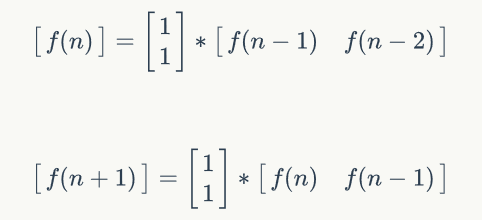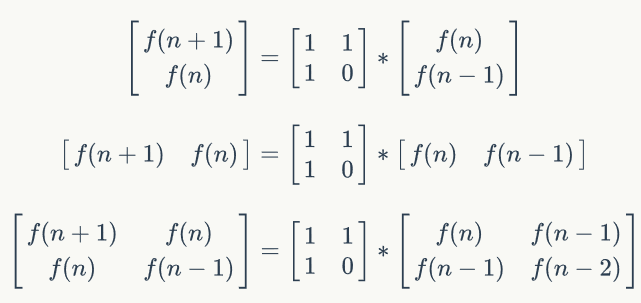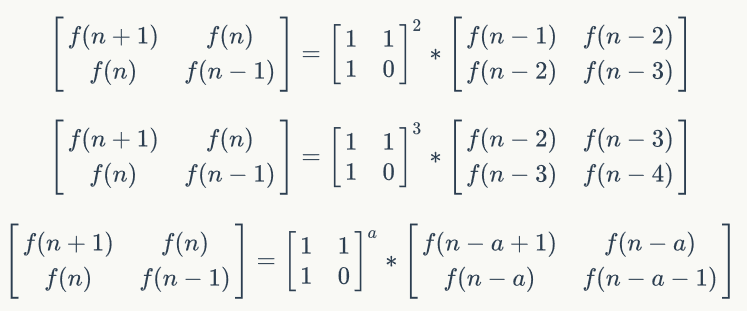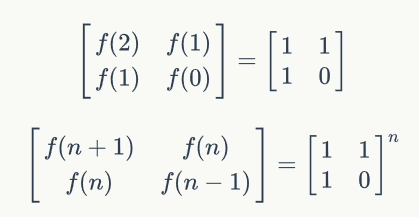2月前 103

递归介绍

• 递：是用来描述方法在何种情况下需要调用自身递下去
• 归：是用来限定方法何时回归，因为程序不可能无限制的走下去

f(x，y) = x * f(x, y - 1)
f(x, 1) = x

递归的缺点

/**
* @param x 底数
* @param y 指数
* @return x的y次幂
*/
long power(int x, int y) {
long res = 1;
for(int i = 1; i <= b; i++) {
res *= a;
}
return  res;
}

/**
* @param x 底数
* @param y 指数
* @return x的y次幂
*/
long power(int x, int y) {
if(y == 1) {
return x;
} else {
return x * power(x, y-1)
}
}

整数快速幂

/**
* @param x 底数
* @param y 指数
* @return x的y次幂
*/
long power(int x, int y) {
long res = 1;
while(y) {
if(y&1) {
res = res * x;    //如果二进制最低位为1，则乘上x^(2^i)
}
x = x * x;            //将x平方
y >>= 1;            　//右移 相当于n/2
}
return res;
}

1. res = 1
2. while循环，此时y = 21 = 10101(二进制)
3. if(y&1),y = 10101(二进制),判断结果为true（二进制的最低位是1）
4. res = res * x = 1 * 2 = 2
5. x = x * x = 2 * 2 = 2^2
6. y >>= 1 = 1010(二进制)
7. while循环，此时y = 10101(二进制)
8. if(y&1),y = 1010(二进制),判断结果为false（二进制的最低位是0）
9. x = x * x = 2^2 * 2^2 = 2^4
10. y >>= 1 = 101(二进制)
11. while循环，此时y = 101(二进制)
12. if(y&1),y = 101(二进制),判断结果为true（二进制的最低位是1）
13. res = res * x = 2 * 2^4 = 2^5
14. x = x * x = 2^4 * 2^4 = 2^8
15. y >>= 1 = 10(二进制)
16. while循环，此时y = 10(二进制)
17. if(y&1),y = 10(二进制),判断结果为false（二进制的最低位是0）
18. x = x * x = 2^8 * 2^8 = 2^16
19. y >>= 1 = 1(二进制)
20. while循环，此时y = 1(二进制)
21. if(y&1),y = 1(二进制),判断结果为true（二进制的最低位是1）
22. res = res * x = 2^16 * 2^5 = 2^5
23. x = x * x = 2^16 * 2^16 = 2^32
24. y >>= 1 = 0(二进制)
25. while循环结束
26. retrun res,也就是计算结果啦

斐波那契数列（兔子）

x > 2: f(x) = f(x-1) + f(x-2)
x = 2: f(x) = 1
x = 1: f(x) = 1

int fei(int n) {
if(n == 1||n == 2) {
return 1;
}
return fei(n-1) + fei(n-2);
}

斐波那契数列的方程组转化为矩阵计算的推导过程

• f(n) = f(n-1) + f(n-2) 得:
• f(n+1) = f(n) + f(n-1)